# Algebraic fraction facts for kids

Kids Encyclopedia Facts

An algebraic fraction is a fraction where the top and the bottom are algebraic expressions. Two examples of algebraic fractions are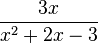$\frac{3x}{x^2+2x-3}$ and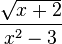$\frac{\sqrt{x+2}}{x^2-3}$.

A rational fraction is an algebraic fraction where the top and the bottom are polynomials.$\frac{3x}{x^2+2x-3}$ is a rational fraction, but$\frac{\sqrt{x+2}}{x^2-3}$ is not. This is because the top has a square root function.

## Operations

### Multiplication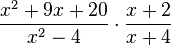$\frac{x^2+9x+20}{x^2-4} \cdot \frac{x+2}{x+4}$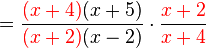$= \frac{{\color{Red}(x+4)}(x+5)}{{\color{Red}(x+2)}(x-2)} \cdot \frac{\color{Red}x+2}{\color{Red}x+4}$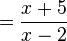$= \frac{x+5}{x-2}$

### Division

Turn the equation into multiplication by flipping one of the fractions. After that, do what is shown above.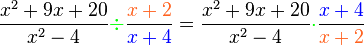$\frac{x^2+9x+20}{x^2-4} {\color{Green}\div} \frac{\color{Orange}x+2}{\color{Blue}x+4} = \frac{x^2+9x+20}{x^2-4} {\color{Green}\cdot} \frac{\color{Blue}x+4}{\color{Orange}x+2}$In Spanish: Fracción algebraica para niños